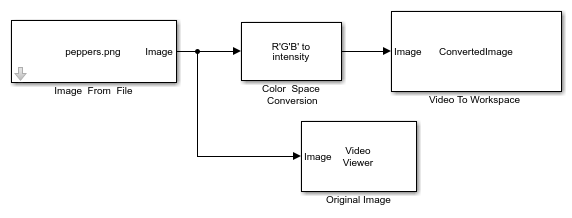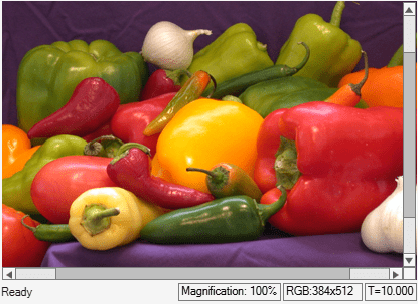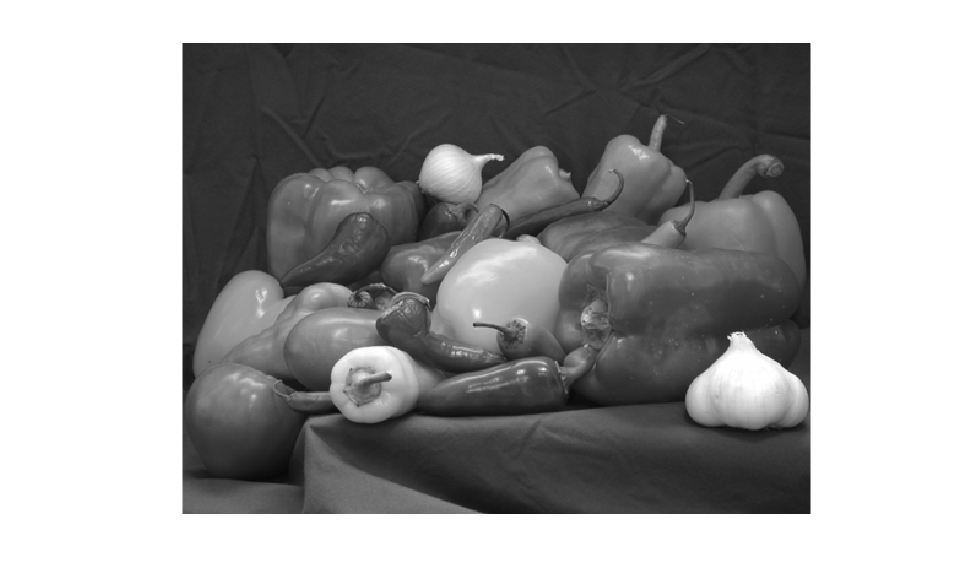Export Image to MATLAB Workspace

This model shows how to export an image from Simulink to MATLAB workspace by using the Video To Workspace block.

Example Model

This model takes a color image as the input, converts it into a gray scale image and exports the converted image to MATLAB workspace.

modelname='ex_blkvideotoworkspace.slx';
open_system(modelname);To convert the original image into gray scale, set the Conversion parameter of Color Space Conversion block to R'G'B to intensity. The original image is of size 384-by-512-by-3 and the gray scale image output from the Color Space Conversion block is of size 384-by-512.

Export the converted image to MATLAB workspace as a variable named ConvertedImage using the Video To Workspace block. You can display the original image using the Video Viewer block.

Simulate and Display Results

sim(modelname);The Video To Workspace block exports the converted image as a video with two identical frames and is of size 384-by-512-by-2. Use the imshow function to display the first frame in the video.

imshow(ConvertedImage(:,:,1))